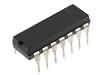7412             TRIPLE 3-INPUT NAND GATE             POSITIVE LOGIC

The 7412 IC package contains three independent positive logic, 3 input NAND GATES. Pins 14 and 7 provide power for all three logic gates.

7412
74LS12 = Low Power Schottkey
74HCT12 = High SpeedA*B*C = X
0*0*0=1
0*0*1=1
0*1*0=1
0*1*1=1
1*0*0=1
1*0*1=1
1*1*0=1
1*1*1=0

Outputs of one gate can be connected to inputs of another within the same chip or to another chip as long as they share the same ground. The figure to the left illustrates a basic circuit showing how to wire inputs and using LEDs to display outputs.

NAND GATE Logic-Rules:
The same basic rules apply for the triple input NAND gate as in other more common 2-input variants.

If the three inputs are labeled A, B, and C and the output = X, then the states can be modeled by the chart on the left.